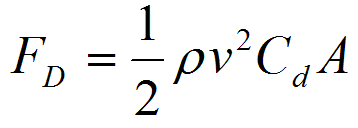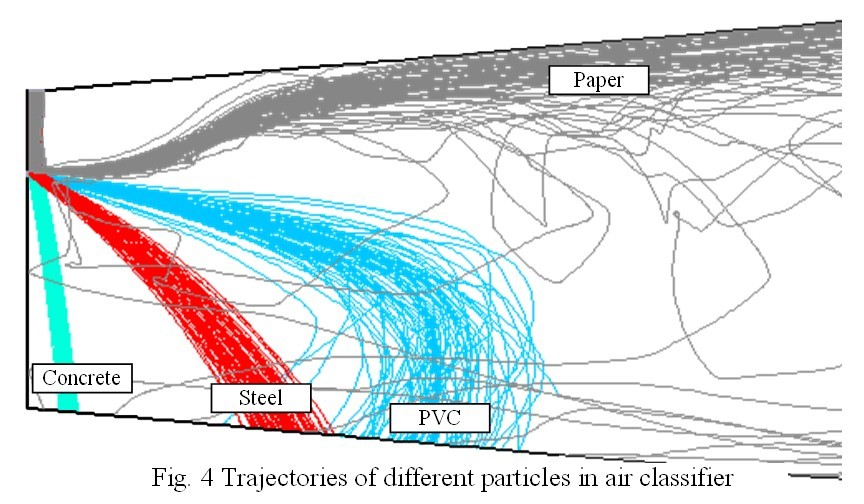# Garbage sorting

#### Finally we want to tell about nice problem that was solved several years ago in Finnish-Russian study group that took place in Lappeenranta. It is a good example of usefulness of such event for industrial company.

The problem proposed for consideration in ESGI-89 by BMH Corporation was to develop the mathematical model and perform simulations of the waste handling process in air classifier in order to evaluate the sorting precision.

The air classifier (Fig. 1) is the special device for sorting the waste particles using the air stream. In waste handling process air classification ensures the high quality of Solid Recovered Fuel (SRF). Sorting precision is that the inert materials like concrete and stones (heavy material) are sorted out, that is the heavy fraction drops to certain area in the air classifier when the light fraction is blown forward over the flap.Fig. 1 General appearance and operating principle of air classifier

The particles of every distinctive material have specific density as well as size and shape distribution (Fig 2). All these factors need to be taken into account in mathematical model.Fig. 2 Shredded waste that is transported into air classifier

The air flow inside classifier was simulated by solving Reynolds averaged Navier-Stokes (RANS) equations. Classical k-ε model [e.g. see Wilcox] was used for closure of RANS system.
The most challenging part of the model is the simulation of the interaction between particles and air flow. It is known [e.g. see Massey, Ward-Smith] that drag force on the particle moving in the fluid is described by the following equation:Here FD is the drag force which is codirectional with the flow velocity, ρ is mass density of the fluid; v is the speed of the particle relative to the fluid; A is the reference particle area and Cd is drag coefficient that depends on Reynolds number as well as particle shape. Following [Haider, Levenspiel] we describe the shape of the particle by one parameter called sphericity that is the ratio between the surface of the sphere having the same volume as the particle and the actual surface of the particle. In our model Cd of a particle is computed as function of particle sphericity and local Reynolds number by relation given in [Haider, Levenspiel].
Also it is possible to take into account the influence of particles onto the air flow by adding supplementary source term into the momentum equation of the Navier-Stokes system. It is particularly important for regimes with large charge of the waste into air classifier.
The model was realized using Ansys Fluent code. Both 2D and 3D were considered.
Now we give some simulation results. The air velocity in the input region of air classifier is given in Figure 3.Fig. 3 Air velocity field in the input region of air classifier

The trajectory examples for particles of different materials are given in Figure 4.As it seen from Figure 4 the different materials are tolerably separated by air classifier.
The developed simulation methodology provides satisfactory results and after fine tuning by physical experiments it can be recommended for industrial use.

References

Wilcox D.C. Turbulence Modeling for CFD. DCW Industries Inc., 1998

Massey B.,Ward-Smith J. Mechanics of Fluids, 8th Edition. Taylor&Francis, London, New York (2006).

Haider A., Levenspiel O.: Drag coefficient and terminal velocity of spherical and nonspherical particles Powder Technology. 58, 1989, 63-70.